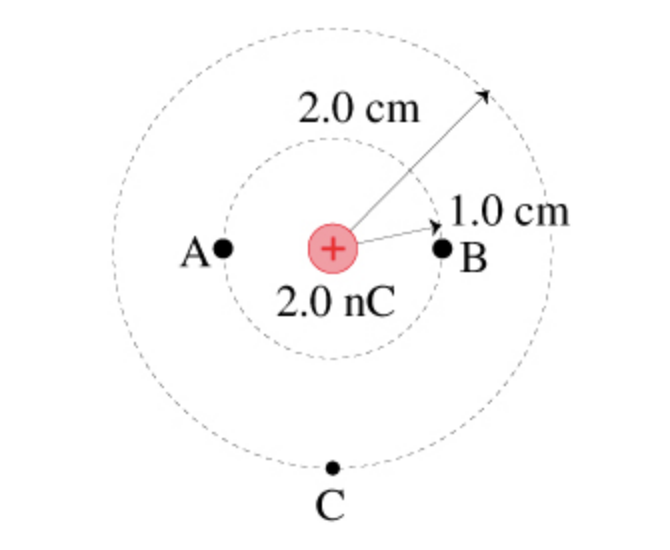# Problem: a. What is the potential difference ΔVAB?b. What is the potential difference ΔVBC?

###### FREE Expert Solution

Potential:

$\overline{){\mathbf{V}}{\mathbf{=}}\frac{\mathbf{k}\mathbf{q}}{\mathbf{r}}}$

a.

Potential difference:

83% (250 ratings)###### Problem Detailsa. What is the potential difference ΔVAB?

b. What is the potential difference ΔVBC?